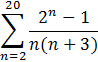### Sample Problem

Use sigma notation to write the sum:#### SolutionNumerator: 3, 7, 15, 31…1048575→2n-1 where n=2, 3, 4…20

Denominator: 2∙5, 3∙6, 4∙7, 5∙8…20∙23→n∙ (n+3) where n=2, 3, 4…20

Therefore since n starts with 2 and ends with n=20, the sigma notation for this sum=